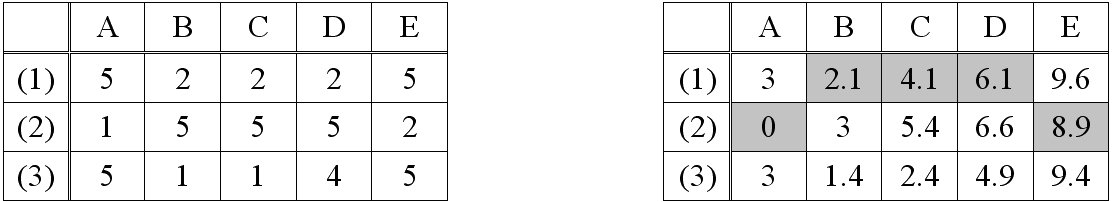Figure 1: A sample isotropic cost grid (left) and the corresponding ACS (right) for movement from cell A(2) to cell E(2), based on Queen's moves. The cells forming the LCP from A(2) to E(2) are marked by a grey background. Examples for cost calculations: The costs of the horizontal movement A(2) to B(2) are (1+5)/2; the costs of the diagonal movement from A(2) to B(1) amount to √2*((1+2)/2). The costs of movement from A(2) via B(1) to C(1) is the sum √2*((1+2)/2) + (2+2)/2. Image credit: I. Herzog.